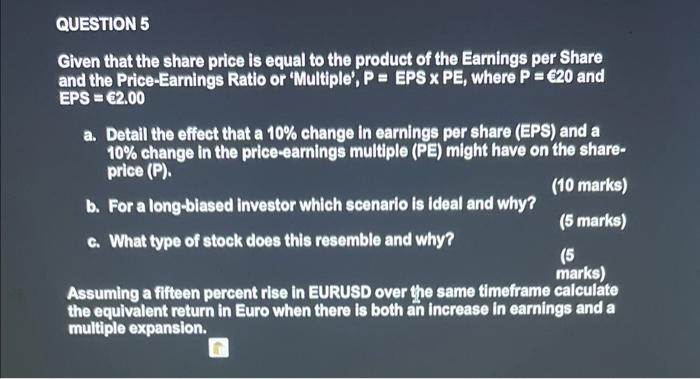Home / Expert Answers / Finance / question-5-given-that-the-share-price-is-equal-to-the-product-of-the-earnings-per-share-and-the-pri-pa684

# (Solved): QUESTION 5 Given that the share price is equal to the product of the Earnings per Share and the Pri ...QUESTION 5 Given that the share price is equal to the product of the Earnings per Share and the Price-Earnings Ratio or 'Multiple', $$P=E P S \times P E$$, where $$P=620$$ and EPS $$=\mathbf{6 2 . 0 0}$$ a. Detail the effect that a $$10 \%$$ change in earnings per share (EPS) and a $$10 \%$$ change in the price-earnings multiple (PE) might have on the shareprice $$(\mathrm{P})$$. b. For a long-biased investor which scenario is ideal and why? (10 marks) c. What type of stock does this resemble and why? (5 marks) (5) Assuming a fifteen percent rise in EURUSD over the same timeframe calculate the equivalent return in Euro when there is both an increase in earnings and a multiple expansion.

We have an Answer from Expert